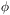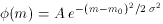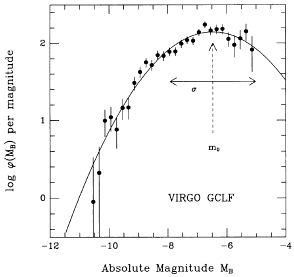4.2 Method

Detailed descriptions of the present-day use of globular cluster luminosity functions (or GCLFs) can be found in Hanes and Whittaker (1987), Harris (1988a), and Harris et al. (1991) along with their results for the distance scale and H0. The basic ideas are illustrated in Figure 7, which is a plot of the GCLF (the relative number(m) of globular clusters as a function of magnitude m) for the Virgo giant ellipticals. The functional shape of(m) is characterized by two simple parameters: the magnitude level or turnover point (m0) where the population of clusters reaches a maximum; and the dispersion ((m) or standard deviation of the distribution. As an empirical curve, the observations show that a Gaussian or log-normal function(6)

(where A is a normalization factor representing the peak population of globular clusters in the galaxy) does an excellent job of describing the GCLF in all galaxies studied to date. The log-normal fitting function gives a convenient way to parameterize the data and to intercompare the GCLFs from different galaxies. For the nearest rich systems of galaxies containing giant ellipticals (the Virgo and Fornax clusters) the deepest limits achieved at present reach to just about 1fainter than the turnover (Harris et al. 1991).Figure 7. The luminosity function for the globular clusters in four Virgo ellipticals combined (NGC 4365, 4472, 4486, 4649), from Harris et al. (1991). This composite GCLF was constructed from a total sample of about 2000 clusters brightes than B = 26.2 (the vertical scale units shown are arbitrary). Hereis the relative number of clusters per unit magnitude interval, plotted against absolute magnitude MB assuming d(Virgo)17 Mpc. A Gaussian interpolation curve is superimposed, with a ``trnover'' or peak indicated by the dashed arrow (m0), and a standard deviation= 1.45 mag.

The actual use of the GCLF method, in its simplest form, goes as follows. The `standard candle' is essentially the magnitude of the turnover, m0. The goal in observing any distant galaxy is then to obtain photometry of its halo clusters which reaches sufficiently faint to estimate m0 accurately. In practice, the GCLF is normally found as the residual excess of starlike objects (see Figure 6), after a background LF for the field has been subtracted and photometric detection incompleteness at the faintest levels has been accounted for. By fitting an analytic interpolation function (such as the Gaussian model above) to the observed data, or else by using a maximum-likelihood fit to the total observed LF (GCLF plus background), the curve parameters (A, m0,) and their internal uncertainties can be estimated (van den Bergh et al. 1985; Pritchet and van den Bergh 1985b; Hanes and Whittaker 1987; Harris et al. 1991). Adopting an absolute magnitude M0(turnover), and any necessary correction for foreground reddening, immediately yields the distance modulus.

If the limiting magnitude of the photometry goes clearly past the turnover as in the example of Figure 7, both m0 andcan be solved for simultaneously. Ideally, the uncertainty e (m0) would be equal to/N, which for a sample size N of several hundred or more clusters should be ± 0.1 mag or smaller. In practice, the extra noise introduced by the background LF subtraction and completeness corrections, and the uncertainty initself, lead to best-case results near e (m0)± 0.2 mag for a single galaxy. However, if the limit of the data falls at or a bit short of the turnover, then the fitted solutions for m0 andbecome strongly correlated (Hanes and Whittaker 1987). It is still possible to estimate m0 to a typical precision of ± 0.3 mag, but only by performing a restricted function fit with an assumed, externally-known value of(hence the importance of the basic premise that the GCLF parameters are the same for galaxies of the same type; see Sec. 4.4). An alternative is to adopt the well-defined Virgo GCLF (Figure 7) as a fiducial function and to match any other galaxy to it by adjusting its distance relative to Virgo until a best fit is achieved.

The range of applicability of the GCLF method, and its basic strengths, are readily summarized.

(1) The GCLF works by far the best in giant E galaxies, which contain the largest globular cluster populations (N1000 is observationally feasible in such galaxies). On the other hand, the GCLF method is immensely more difficult to employ in late-type galaxies (Sc, Sd, Irr), and so in most cases it is difficult to match the GCLF distance scale directly with any of the Population I techniques.

(2) Because the clusters are nonvariable objects, they do not require repeated or carefully scheduled time-series observations. Telescope time is thus employed efficiently.

(3) Because it applies to objects in the halos of large galaxies, the method is free of a host of observational problems that affect all Population I standard candles, such as internal reddening differences, crowding and source confusion, and inclination-angle corrections.

(4) The distance range of the primary GCLF method is set by the limiting magnitude at which the turnover M0 is still detectable. With current ground-based capabilities, the limit should be near d50 Mpc (Harris 1988a).